Surface Area
Volume
Cross Sections
Word Problems and Applications
Formulas
100

Find the surface area of the rectangular prism.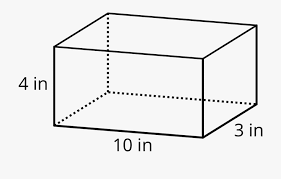164 in2

100

Find the volume of the rectangular prism.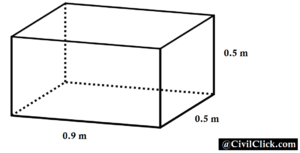0.225 m3

100

The horizontal cross section of a cylinder: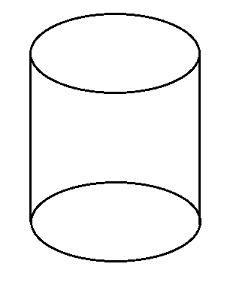Circle

100

A room is 144 ft2. If tile costs \$0.83 per square foot, how much would it cost to put tile in?

\$119.52

100

Name that formula: 1/2 h (b1 + b2)

Area of a trapezoid

200

Find the surface area of the rectangular prism.2.3 m2

200

Find the volume of the rectangular pyramid.224 cm3

200

The vertical cross section of a cylinder:Rectangle

200

A gallon of paint can cover 45 square feet. If a wall is 325 square feet, how many gallons of paint should you buy?

8 gallons

200

Name that formula: 1/2 b h

Area of a triangle

300

Find the surface area of the triangular prism.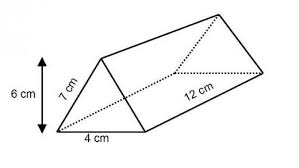240 cm2

300

Find the volume of the triangular prism.144 cm3

300

The vertical cross section of a cone, through its apex: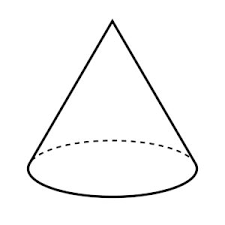Triangle

300

You are filling the rectangular pyramid with sand. How many cubic inches of sand will you need?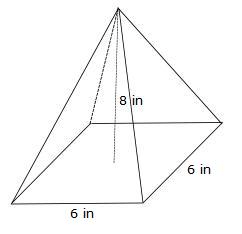96 in3

300

Name that formula: bh

Area of a parallelogram/rectangle/square

400

Find the surface area of the square pyramid.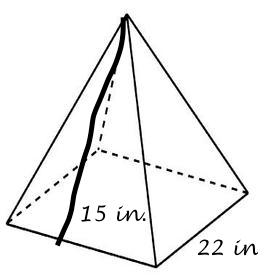1,144 in2

400

Find the volume of the cylinder. Use 3.14 for pi.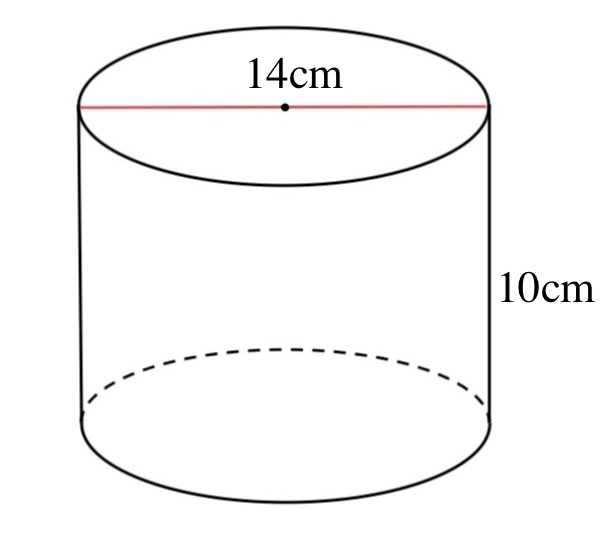1,538.6 cm3

400

What is the largest cross-section that could result from slicing a rectangular prism?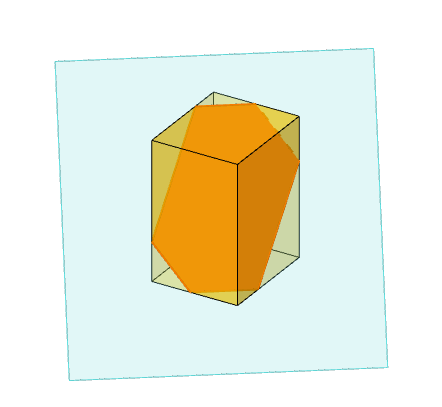Hexagon

400

If it costs \$0.25  per square inch to paint this prism, approximately how much will it cost?\$30

400

Name that formula: 1/3 B h

Volume of a pyramid

500

Find the surface area of the trapezoidal prism.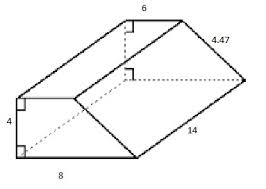506.58 units2

500

Find the volume of the trapezoidal prism.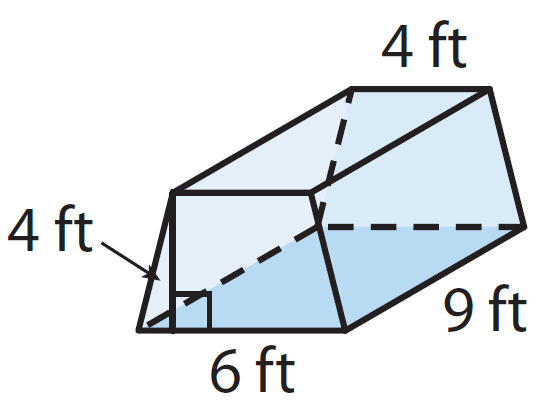180 ft3

500

If a cone was sliced at an angle, what would be the resulting cross-section?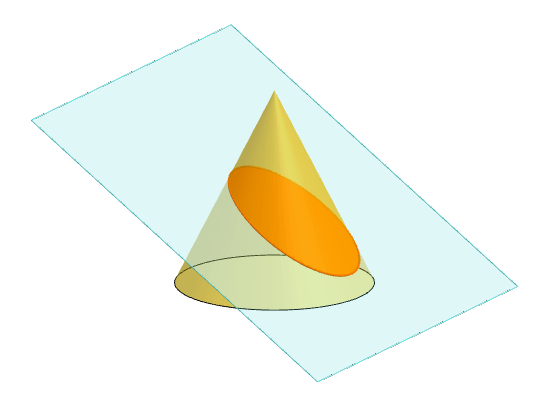Oval (bonus points if you said ellipse)

500

The following prism is being filled with sand. Sand costs \$2.25 per square foot.How much will it cost?

\$405

500

Name that formula: B h

Volume of a prism

Click to zoom